Updated 27/09/2023
In force

Version from: 28/06/2023
Amendments (1)
There is currently no level 2 legal act based on or specifying Article 281.
Search within this legal act

### Article 281 - Calculation of the exposure value

Article 281

Calculation of the exposure value

1.
Institutions shall calculate a single exposure value at netting set level in accordance with Section 3, subject to paragraph 2 of this Article.
2.

The exposure value of a netting set shall be calculated in accordance with the following requirements:

(a)

institutions shall not apply the treatment referred to in Article 274(6);

(b)

by way of derogation from Article 275(1), for netting sets that are not referred to in Article 275(2), institutions shall calculate the replacement cost in accordance with the following formula:

RC = max{CMV, 0}

where:

 RC = the replacement cost; and
 CMV =
(c)

by way of derogation from Article 275(2) of this Regulation, for netting sets of transactions: that are traded on a recognised exchange; that are centrally cleared by a central counterparty authorised in accordance with Article 14 of Regulation (EU) No 648/2012 or recognised in accordance with Article 25 of that Regulation; or for which collateral is exchanged bilaterally with the counterparty in accordance with Article 11 of Regulation (EU) No 648/2012, institutions shall calculate the replacement cost in accordance with the following formula:

RC = TH + MTA

where:

 RC = the replacement cost;
 TH = the margin threshold applicable to the netting set under the margin agreement below which the institution cannot call for collateral; and
 MTA = the minimum transfer amount applicable to the netting set under the margin agreement;
(d)

by way of derogation from Article 275(3), for multiple netting sets that are subject to a margin agreement, institutions shall calculate the replacement cost as the sum of the replacement cost of each individual netting set, calculated in accordance with paragraph 1 as if they were not margined;

(e)

all hedging sets shall be established in accordance with Article 277a(1);

(f)

institutions shall set to 1 the multiplier in the formula that is used to calculate the potential future exposure in Article 278(1), as follows: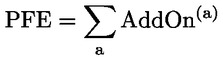where:

 PFE = the potential future exposure; and
(g)

by way of derogation from Article 279a(1), for all transactions, institutions shall calculate the supervisory delta as follows:

δ =+ 1 where the transaction is a long position in the primary risk driver

– 1 where the transaction is a short position in the primary risk driver

where:

 δ = the supervisory delta;
(h)

the formula referred to in point (a) of Article 279b(1) that is used to compute the supervisory duration factor shall read as follows:

supervisory duration factor = E – S

where:

 E = the period between the end date of a transaction and the reporting date; and
 S = the period between the start date of a transaction and the reporting date;
(i)

the maturity factor referred to in Article 279c(1) shall be calculated as follows:

(i)

for transactions included in netting sets referred to in Article 275(1), MF = 1;

(ii)

for transactions included in netting sets referred to in Article 275(2) and (3), MF = 0,42;

(j)

the formula referred to in Article 280a(3) that is used to calculate the effective notional amount of hedging set j shall read as follows: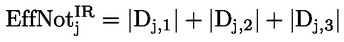where: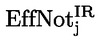= the effective notional amount of hedging set j; and
 Dj,k = the effective notional amount of bucket k of hedging set j;
(k)

the formula referred to in Article 280c(3) that is used to calculate the credit risk category add-on for hedging set j shall read as follows: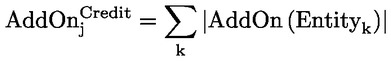where: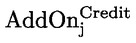= the credit risk category add-on for hedging set j; and
(l)

the formula referred to in Article 280d(3) that is used to calculate the equity risk category add-on for hedging set j shall read as follows: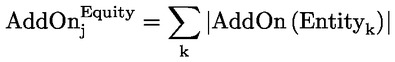where: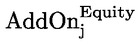= the equity risk category add-on for hedging set j; and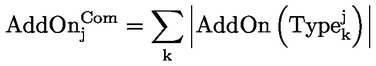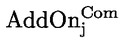= the commodity risk category add-on for hedging set j; and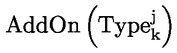= the add-on for the commodity reference type k.